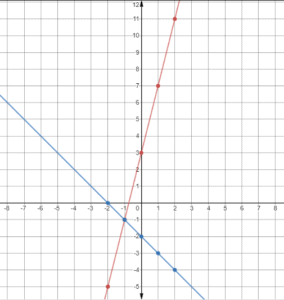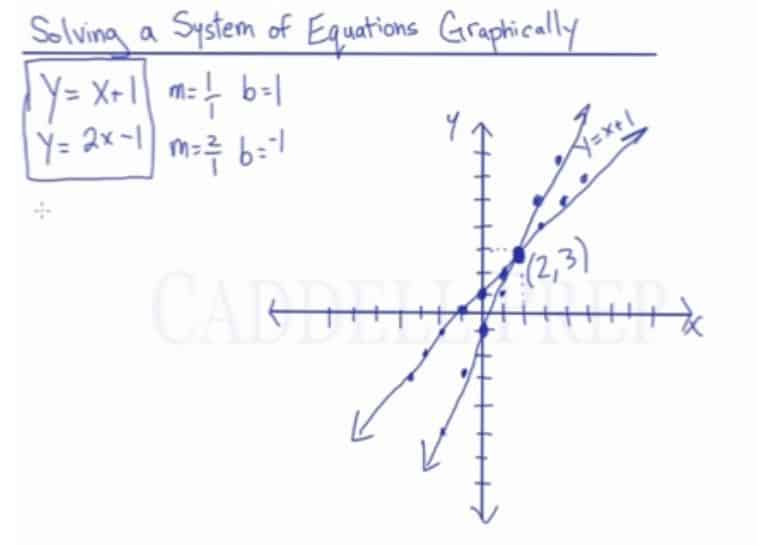Learn how to solve a system of equations graphically by plotting points and finding the point(s) of an intersection. After you finish this lesson, view all of our Algebra 1 lessons and practice problems.
There are two ways to find the point where your lines intersect. After you finish this lesson, view all of our Pre-Algebra lessons and practice problems.

The graphical way to find the point of intersection is to graph the equations by first writing the slope and y-intercept of each line. ( the “m” value and the “b” value. )
The slope is another way of measuring rise over run or the ratio of distances from each point on the line. Start plotting points from each line’s y-intercept, and then plot the point from there using the slope of the line.
When you do this for each line, you get a point of intersection where the coordinates of that point are identical on both lines.
Another way to find the point of intersection is by making a table for the x and y values, and finding the coordinates by plugging each x value into the line equation for both lines.
An example:$y = x + 1$$y = 2x - 1$$y = x + 1$$y-value$$coordinates$$x-value$
1$y = (1) + 1$ 2 (1,2)
2$y = (2) + 1$ 3 (2,3)
3$y = (3) + 1$ 4 (3,4)
4$y = (4) + 1$ 5 (4,5)
5$y = (5) + 1$ 6 (5,6)$y = 2x - 1$$y-value$$coordinates$$x-value$
1$y = 2(1) - 1$ 1 (1,1)
2$y = 2(2) - 1$ 3 (2,3)
3$y = 2(3) - 1$ 5 (3,5)
4$y = 2(4) - 1$ 7 (4,7)
5$y = 2(5) - 1$ 9 (5,9)

So now you find the two identical coordinates from the two tables, and that coordinate would be your point of intersection.

## Examples Solving A System Of Equations Graphically

### Example 1$y = 5x +1$$y = 2x+4$

For$y = 5x +1$For$y = 2x+4$### Example 2$y = 4x+3$$y = -x-2$

For$y = 4x+3$For$y = -x-2$## Video-Lesson Transcript

In this lesson, we will discuss how to solve a system of equations graphically.

A system of equations is two or more equations.

And to solve it, we have to have the value of$x$ and$y$. In order to do that, we have to work on the two given equations. Remember, not just one but both of the equations at the same time.

We’re going to solve the system of equation by using the graph. We will plot the points of the given equations. We will graph them and find the intersection of these two equations.

The intersection of the two equations represents the$x$ and$y$ values.

For example, we have$y = x + 1$
and$y = 2x + 1$

Just a review, the formula for slope is$m = \dfrac{y_1 - y_2}{x_1 - x_2}$ and$b = y$-intercept.

So for$y = x + 1$

slope is$m = \dfrac{1}{1}$
and$b = 1$

Let’s graph this by starting at$b$ or our$y$-intercept.

So we plot$(0, 1)$ coordinates.

Then we move to the right by$1$ point and go up by$1$ point.

Remember, the formula$slope = \dfrac{rise}{run}$?

Then we move to the left by$1$ point and go down by$1$ point.

Then draw a line segment.

This line is the first equation$y = x + 1$

Let’s move on to the next equation:$y = 2x - 1$

The slope here is$m = \dfrac{2}{1}$
and$b = {-1}$

So let’s start at$y$-intercept which is${-1}$.

Let’s plot$(0, -1)$ coordinates.

Then let’s go to the right by$1$ point and go up by$2$ points.

Then let’s move to the left$1$ point and go down by$2$ points.

Now, we can draw a line. This line represents the second equation$y = 2x - 1$Here, we can see that the two lines intersect at$(2, 3)$ coordinates.

Aside from graphing, we can also use a table.